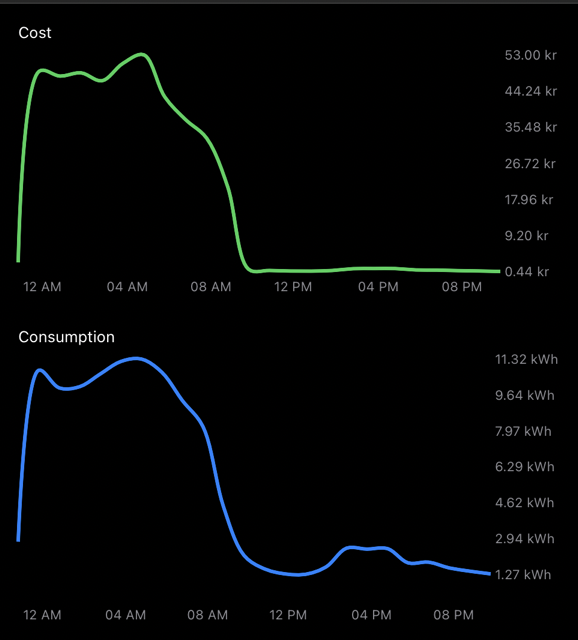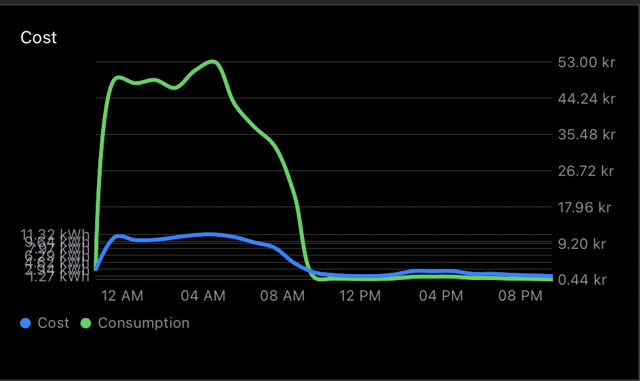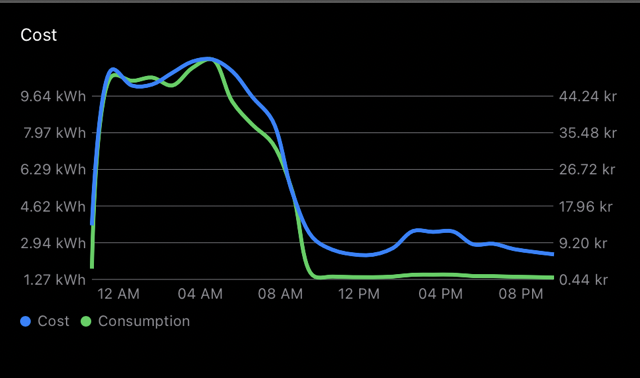# Introduction

In iOS 16, Apple introduced the Swift Charts. With Swift Charts you can make charts such as Bar-, Line- and point charts. See Apple’s documentation for more information and examples. [https://developer.apple.com/documentation/charts]

In this article we are going to create the following chart in Swift UI.In the image above, you’ll notice that we have a line chart with, on the left hand side, one type of data and, on the right hand side, another. In the case above, the left shows the electricity consumption in Kilowatt hours (kWh) and on the right a total cost for the selected hour. The X axis shows the time of the date for the consumption.

## The basics

Lets start with setting up our data first, which we will respresent in SwiftUI. In the example above, we are looking at a 24 hour period of electricity consumption for a home, summarised hour by hour. A similar type of chart you can find in my app Tobbe, where you can login with a Tibber account and show your data.

We’ll start off with a basic playground which will show two charts, one for Consumption and one for Cost. These two we are going to merge later in this article. You can check the gist here: https://gist.github.com/ppeelen/46fa0183f71e761df0c416af0cc0b7f7.

Once you have it running, it should look like:## Axis labels

As you might have noticed, the axis information is not good enough. Lets change that first.

Add the modifier for `chartXAxis` to both Charts:

This will add the X axis labels, which in our case are hour labels for every 4th hour of the given dates.

Now we are going to add the Y axis for both charts, but these are different from each other. One thing they will have in comon is the amount of marks each charts has. We want both charts to have the same amount so they will match up later when we are going to merge them.

Add the following line directly after the `vstack` in the top of the ChartView body, on line 12:

And then add this `chartYAxis` modifier to the cost chart:

And this `chartYAxis` modifier to the consumption chart:

What these will do is add the Y labels to the right side of the chart (trailing) and calculate each of them to their own respecive value by taking the max and min values into account.

Now your chart should look like:## Merging the two

Now that we have two charts we know what data we want to show and how each of them should look. Now lets make one chart of them. We can merge them by moving the second LineMark for the consumption chart, inside the cost chart directly after the first line mark. So go ahead and move that one for now.

When you have move it, you need to let the chart know that these are two different data sets. You can do this by adding the following modifier to the first LineMark:

and this modifier to the consumption LineMark:

Now we need move in the labels for the Y axis too, but we also need to modify them a little. Instead of moving the Y axis labels we’ve created, lets add these instead to the already existing `chartYAxis` modifier for the `Costs` chart:

Now, we can remove the second chart so we only have one chart left. Your `body` for `ChartView` should now look like this:

Last, but not least… lets decrease the height for `MainView` to 250 from 500.

Now if you run the code, the result should look like this:but wait… this looks messed up! I’ll tell you why:

## Fixing the messed up chart

The reason things look messed up now is because the chart’s max value is now at ~53, which is the highest value of the cost per hour. However, the kWh consumption never gets this high. The Chart doesn’t understand that these are two different types of data, it is only concerned about the value… so we can’t use these values. There is a way to solve this though.

Since both the left hand side and the right hand side need to have the same types of values, we could calculate the values to percentage of their respective max values, since that could be the common dinominator here.

Add the following directly after the `strideBy` towards the top of `ChartView`:

This is merly getting the highest and lowest cost values and highest and lowest consumption values. Now change the value of `Price` in the first LineMark to:

and change the value of `Consumption` in the second LineMark to:

Now we need to update the Y axis, since the values won’t match up. You can replace the `chartYAxis` with the following code:

So, what are we doing above? We start off by adding a default stride, one that works for both LineMarks, which goes from 0 to 1 and is offset by the amount of marks we want to make. We use this `defaultStride` for both Y axis (left and right).

When we create each Y axis marks, we then calculate the value of each mark by using the `costsStride` and `consumptionStride` and add our own custom label instead. Now, lets re-run the code and see the result:As you can see, both LineMarks use the full size of the chart, but each side has their own value.

Now, the only thing left to do is adding the scales in the correct order. Right now they show `Cost` and `Consumption`, but they should be the other way around. You can add the following modifier to the Chart to change the order:

This is the final result:Here is the final code for you to try out in a playground: